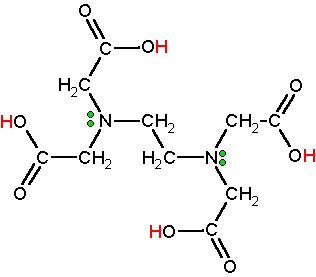# What is the role of buffer solution in complexometric titrations?

Apr 9, 2014

The buffer adjusts the pH to ensure that the reaction goes to completion.

#### Explanation:

A complexometric titration uses the formation of a coloured complex to indicate the endpoint.

EDTA, often written as $\text{H"_4"Y}$, is a common ligand in complexometric titrations.

It has four carboxyl groups and two amine groups that can act as electron pair donors (Lewis bases).EDTA is often used as the disodium salt, $\text{Na"_2"H"_2"Y}$.

It reacts with many metal ions to form a complex:

${\text{M"^(2+) + "H"_2"Y"^"2-" ⇌ "MY"^"2-" + "2H}}^{+}$

The complex has the structureCarrying out the reaction in a basic buffer solution removes the${\text{H}}^{+}$ as it is formed.

This moves the position of equilibrium to the right and favours formation of the complex (Le Châtelier's Principle).

Also, for EDTA, $p {K}_{a 4}$ = 10.26.

Thus, if the solution is buffered to about pH 10.3, most of the EDTA will exist as $\text{Y"^"4-}$ ions.

The metal ions will not have to remove the hydrogen ions from $\text{H"_2"Y"^"2-}$.

They can react directly according to the equation

$\text{M"^(2+) + "Y"^"4-" → "MY"^"2-}$

Above pH 10.3, most metal ions react quantitatively with EDTA.